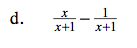### Home > A2C > Chapter Ch6 > Lesson 6.1.2 > Problem6-30

6-30.
1. Perform the indicated operation to simplify each of the following expressions. In some cases, factoring may help you simplify. Homework Help ✎

1.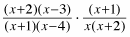2.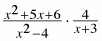3.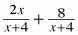4.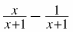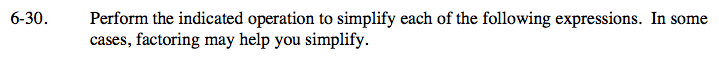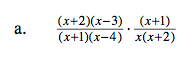$\frac{\textit{x}-3}{\textit{x}(\textit{x}-4)}$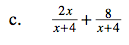Try factoring after adding.

2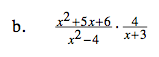$\frac{(\textit{x}+2)(\textit{x}+3)}{(\textit{x}+2)(\textit{x}-2)}\cdot \frac{4}{\textit{x}+3}$